# SSC Quantitative Aptitude Questions (Day-81)

Dear Aspirants, you can find the Quantitative Aptitude questions with detailed explanations for the SSC exams. Nowadays the competitive level of the exam has been increasing consistently. Due to the great demand for the government job, the level of the toughness reached greater. Candidates have to enhance the preparation process in order to drive in the right path. It doesn’t need to clear the prescribed cutoff. You must have to score good marks more than the cut off marks to get into the final provisional list. Here we have updating the Quantitative Aptitude questions with detailed explanations on a daily basis. You can practice with us and measure your level of preparation. According to that you can sculpt yourself in a proper way. SSC aspirants kindly make use of it and grab your success in your career.

Start Quiz

1) If  a/3 = 1- 3/a ; then what is the value of a5?

(a) – 81

(b) 148

(c) – 243

(d) 227

2)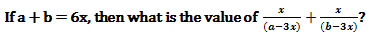(a) 1

(b) 0

(c) 2

(d) – 1

3) If x3 + 6x2 + 12x = 19, then what is the value of x3?

(a) 8

(b) 27

(c) – 1

(d) 1

4)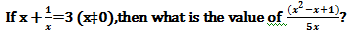(a) 2/5

(b) 3/5

(c) 4/5

(d) 1/5

5) If x2 + 16 = -4x, then what is the value of x3 – 64?

(a) 128

(b) 0

(c) 64

(d) 256

6) PQRS is a rectangle, A, B, C and D are the mid points of sides PQ, QR, RS and PS respectively. If area of ΔPQR is 48 cm2, then what is the area (incm2)  of ΔBCD?

(a) 24

(b) 6

(c) 16

(d) 12

7) If PA is the median of the triangle PQR and G be the centroid, then what is the ratio of (PA + GA) : (PG – GA)?

(a) 4 : 1

(b) 3 : 1

(c) 2 : 1

(d) 3 : 2

8) In a ΔPQR, PD is the median and G is centroid. If PG = 24 cm, then what is the length (in cm) of PD?

(a) 48

(b) 36

(c) 60

(d) 72

9) The tangents drawn at the point P and Q of a circle centered at O meet at A. If ∠POQ = 120°, then what is the ratio of ∠PAQ : ∠PAO?

(a) 2 : 3

(b) 4 : 1

(c) 2 : 1

(d) 5 : 2

10) ABCD is a trapezium, such that AB = CD and AD||BC. AD = 10 cm and BC = 18 cm. If the area of ABCD is 70 cm2, then what is the value (in cm) ofCD?

(a) 5

(b) √29

(c) √41

(d) 6

a/3 + 3/a = 1

then (a/3)3 = –1

a³ = – 27

a = –3

a⁵ = –243

Let x = 1

a = 4   & b = 2

put these values in given equation,

= 1/ ((4 –3)) + 1/(2 –3)

= 1/1  – 1/1

= 0

x³ + 6x² + 12x + 8 = 27

(x + 2)³ = 3³

(x + 2) = 3

x = 1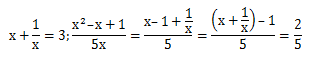x2 +4x + 16 = 0 →  (1)

eqn. (1) × 4 ⇒ 4x2 + 16x + 64 = 0 → (ii)

eqn. (1) Xx ⇒ x3 +4x2 + 16x = 0→ (iii)

eqn. (iii) – eqn .(i)

x3 + 4x2 +16x =0

4x2 +16x + 64 = 0

x3 – 64 = 0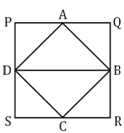Area of ∆BCD = ¼ Area of PQRS

= ½ Area of ∆PQR

= 48/2

= 24 cm²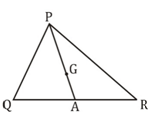Let PA = 3

Then PG = 2        (∵ G is centroid hence PG:GA= 2:1)

GA = 1

Then

= (PA + GA) : (PG – GA)

= (3 + 1) : (2 – 1)

= 4 : 1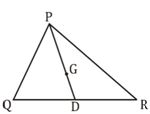If PG = 24 cm

Then, GD = 12 cm

PD = 36 cm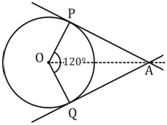∠PAQ= 120/2= 600

∠PAO = 300

∠PAQ : ∠PAO = 2 : 1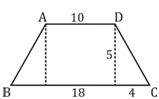Area of trapezium = ½ (sum of parallel sides) × height

70 = ½ (10 + 18) × height

140 = 28 × height

Height = 5 cm

CD =  √(25+16)

=√41 cm

 Check Here to View SSC CGL / CHSL 2021 Quantitative Aptitude Questions Day – 80 Day – 79 Day – 78